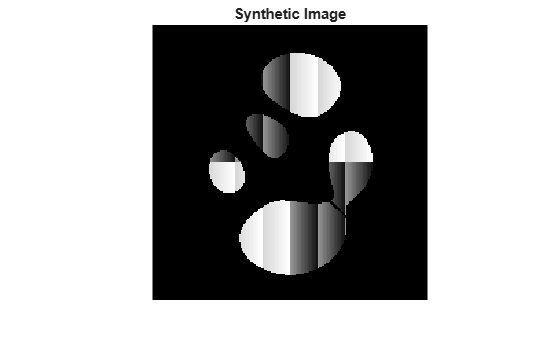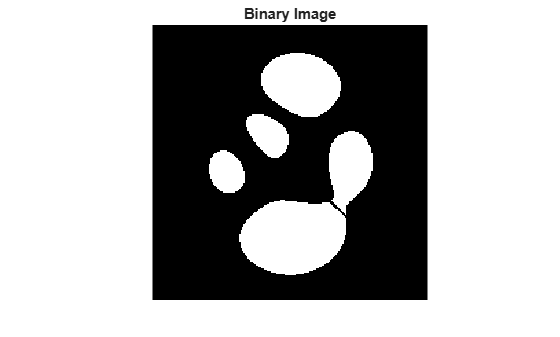# Measuring Regions in Grayscale Images

This example shows how to measure properties of objects in a grayscale image. To accomplish this, first segment the grayscale image to get a binary image of objects. Then, use regionprops to analyze the original grayscale pixel values corresponding to each object in the binary image.

### Step 1: Create Synthetic Image

Use a helper function, `propsSynthesizeImage`, to create a grayscale image that contains five distinct regions.

```I = propsSynthesizeImage; imshow(I) title('Synthetic Image')```### Step 2: Create a Binary Image

Segment the grayscale image by creating a binary image containing the objects in the image.

```BW = I > 0; imshow(BW) title('Binary Image')```### Step 3: Calculate Object Properties Using Pixel Values of Grayscale Image

The `regionprops` function supports several properties that can be used with grayscale images, including 'WeightedCentroid', 'MeanIntensity', 'MinIntensity', and 'MaxIntensity'. These properties use the original pixel values of the objects for their calculations.

For example, you can use `regionprops` to calculate both the centroid and weighted centroid of objects in the image. Note how you pass in the binary image (`BW`) containing your objects and the original grayscale image (`I`) as arguments into `regionprops`.

`s = regionprops(BW,I,{'Centroid','WeightedCentroid'});`

To compare the weighted centroid locations with the unweighted centroid locations, display the original image and then, using the `hold` and `plot` functions, superimpose the centroids on the image.

```imshow(I) title('Weighted (red) and Unweighted (blue) Centroids'); hold on numObj = numel(s); for k = 1 : numObj plot(s(k).WeightedCentroid(1), s(k).WeightedCentroid(2), 'r*') plot(s(k).Centroid(1), s(k).Centroid(2), 'bo') end hold off```### Step 4: Calculate Custom Pixel Value-Based Properties

You can use the 'PixelValues' property to do custom calculations based on the pixel values of the original grayscale image. The 'PixelValues' property returns a vector containing the grayscale values of pixels in a region.

As an example, calculate the standard deviation of each region.

```s = regionprops(BW,I,{'Centroid','PixelValues','BoundingBox'}); imshow(I) title('Standard Deviation of Regions') hold on for k = 1 : numObj s(k).StandardDeviation = std(double(s(k).PixelValues)); text(s(k).Centroid(1),s(k).Centroid(2), ... sprintf('%2.1f', s(k).StandardDeviation), ... 'EdgeColor','b','Color','r'); end hold off```This figure shows the standard deviation measurement superimposed on each object in the image. You can also view the results in other ways, for example as a bar plot showing the standard deviation by label number.

```figure bar(1:numObj,[s.StandardDeviation]) xlabel('Region Label Number') ylabel('Standard Deviation')```You can use the plot to determine how to partition the data. For example, the following code identifies objects with a standard deviation lower than 50.

```sStd = [s.StandardDeviation]; lowStd = find(sStd < 50); imshow(I) title('Objects Having Standard Deviation < 50') hold on for k = 1 : length(lowStd) rectangle('Position',s(lowStd(k)).BoundingBox,'EdgeColor','y'); end hold off```